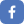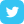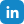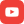R

Techieventures Technologies is the best training institute for R analytics/Programming in BTM layout. R is both a programming language and a free software for analytics and graphics. It runs on pretty much any operating system, and is widely used among statisticians and data miners for data analysis and presentations. Our Course is more focused on providing the best R analytics training.

• #### Introduction to R

• Introduction to R
• Why use R
• History Of R
• Interfaces of R
• Running R Program
• R Commands & Packages
• Some Simple Math
• #### Vector

• Introduction to Vector
• Types of Vector
• Creating vector
• Naming Vectors
• Viewing Vector Objects
• Manipulating Vector
• Vector Maths
• Selecting & Displaying Parts of Vector
• Sorting and Rearranging Vectors
• Returning Logical Values from Vectors
• Missing Values
• #### Matrix

• Introduction to Matrix in R
• Matrix Creation
• Naming Matrix
• Selecting & Displaying Parts of Matrix
• Sorting and Rearranging Matrix
• Accessing Values of Matrix
• Matrix Calculation
• Matrix Manipulation
• Conversion of Vector to Matrix
• #### R- Data Types & Function

• Character Data Type
• Numeric Data Type
• Integer Data Types
• Functions
• SUBSTR
• SUB
• PASTE
• PASTE0
• NCHAR
• TOUPPER
• TOLOWER
• BUILTINS
• OPTIONS
• Logical Data Type
• Complex Data Type
• #### Variable Types & List

• Atomic Variable
• Recursive Variable
• Naming List
• Accessing List Values
• Conversion from Vector to List
• #### Creating R Functions

• Introduction to Functions
• Scope of Variable
• Function Arguments
• Return Values
• do.call
• #### Factor

• Introduction
• Defining Levels
• Comparing Factor
• Types of Factor
• Creating Ordinal Factor
• #### Reading Data into R

• Excel Data
• Data from Other Statistical Tools
• R Binary Files
• Data Extraction from Websites
• #### Charts & Graphs in R

• Types of Plots
• Lattice Plots
• Lattice Scatter Plots
• Bar Chart
• Box Plot
• Histograms
• ggplot - Introduction
• Layers of ggplot
• Using Geoms and Stats
• Adding Facets, Scales, And Options
• Changing Options
• If and Else
• Switch
• If else
• FOR Loops
• Looping Without Loops
• Constructing a FOR Loop
• Calculating values in a FOR Loop
• Looping Through Values
• Looping Without Loops
• WHILE Loops
• Controlling Loops
• Hunting Bugs
• Creating Custom Message
• Normal Distribution
• Binomial Distribution
• Poisson Distribution
• Other Distribution
• Random Numbers & Sampling
• Two-Sample t-Test With Equal Variance
• Two-Sample t-Test With Unequal Variance
• Mann - Whitney Test
• Paired T- And U- Test
• Correlation and Covariance
• Analysis Of Variance (ANOVA)
• One-Way ANOVA
• Two-Way ANOVA
• Extracting Means & Summary Statistics
• Complex ANOVA Models
• Simple Linear Regression
• Multiple Linear Regression
• Curvilinear Regression
• Logistic Regression
• Poisson Regression
• Survival Analysis
• Splines
• Decision Tree
• Random Forests
• Autoregression Moving Average
• VAR
• GARCH
• K-Means
• PAM
• Hierarchical Clustering

#### Other CoursesLike us!Follow us!Follow us!subscribe !